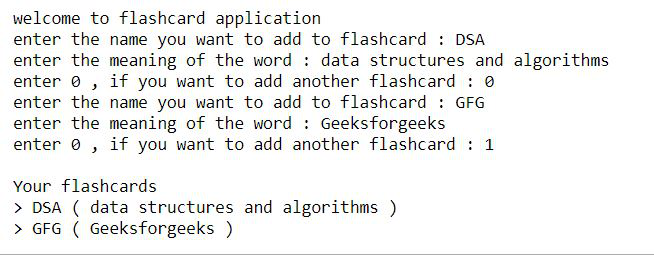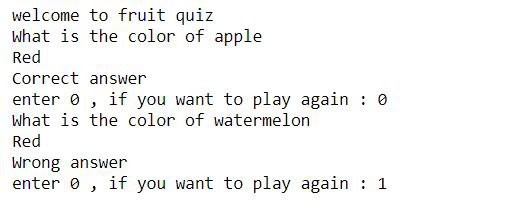Open In App
Related Articles

# Python program to build flashcard using class in Python

In this article, we will see how to build a flashcard using class in python. A flashcard is a card having information on both sides, which can be used as an aid in memoization. Flashcards usually have a question on one side and an answer on the other. Particularly in this article, we are going to create flashcards that will be having a word and its meaning.

Let’s see some examples of flashcard:

Example 1:

Approach :

• Take the word and its meaning as input from the user.
• Create a class named flashcard, use the __init__() function to assign values for Word and Meaning.
• Now we use the __str__() function to return a string that contains the word and meaning.
• Store the returned strings in a list named flash.
• Use a while loop to print all the stored flashcards.

Below is the full implementation:

## Python3

 `class` `flashcard:``    ``def` `__init__(``self``, word, meaning):``        ``self``.word ``=` `word``        ``self``.meaning ``=` `meaning``    ``def` `__str__(``self``):``      ` `        ``#we will return a string``        ``return` `self``.word``+``' ( '``+``self``.meaning``+``' )'``      ` `flash ``=` `[]``print``(``"welcome to flashcard application"``)` `#the following loop will be repeated until``#user stops to add the flashcards``while``(``True``):``    ``word ``=` `input``(``"enter the name you want to add to flashcard : "``)``    ``meaning ``=` `input``(``"enter the meaning of the word : "``)``    ` `    ``flash.append(flashcard(word, meaning))``    ``option ``=` `int``(``input``(``"enter 0 , if you want to add another flashcard : "``))``    ` `    ``if``(option):``        ``break``        ` `# printing all the flashcards``print``(``"\nYour flashcards"``)``for` `i ``in` `flash:``    ``print``(``">"``, i)`

Output:Time Complexity : O(n)

Space Complexity : O(n)

Example 2:

Approach :

• Create a class named flashcard.
• Initialize dictionary fruits using __init__() method.
• Now randomly choose a pair from fruits using choice() method and store the key in variable fruit and value in variable color.
• Now prompt the user to answer the color of the randomly chosen fruit.
• If correct print correct else print wrong.

## Python3

 `import` `random` `class` `flashcard:``    ``def` `__init__(``self``):``      ` `        ``self``.fruits``=``{``'apple'``:``'red'``,``                     ``'orange'``:``'orange'``,``                     ``'watermelon'``:``'green'``,``                     ``'banana'``:``'yellow'``}``        ` `    ``def` `quiz(``self``):``        ``while` `(``True``):``          ` `            ``fruit, color ``=` `random.choice(``list``(``self``.fruits.items()))``            ` `            ``print``(``"What is the color of {}"``.``format``(fruit))``            ``user_answer ``=` `input``()``            ` `            ``if``(user_answer.lower() ``=``=` `color):``                ``print``(``"Correct answer"``)``            ``else``:``                ``print``(``"Wrong answer"``)``                ` `            ``option ``=` `int``(``input``(``"enter 0 , if you want to play again : "``))``            ``if` `(option):``                ``break` `print``(``"welcome to fruit quiz "``)``fc``=``flashcard()``fc.quiz()`

Output:Time Complexity : O(1)

Space Complexity : O(1)